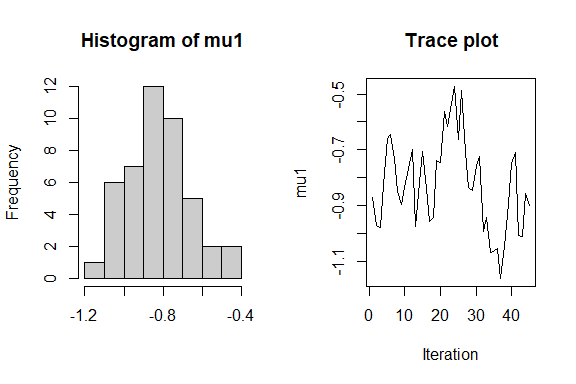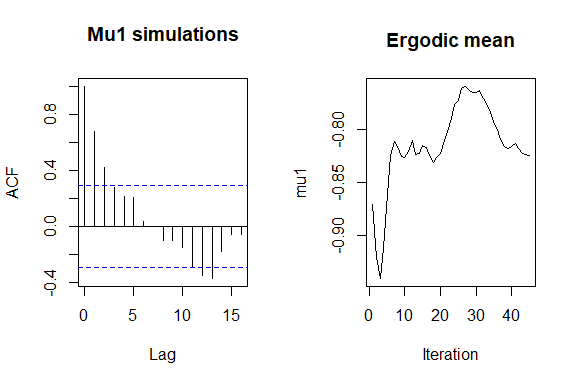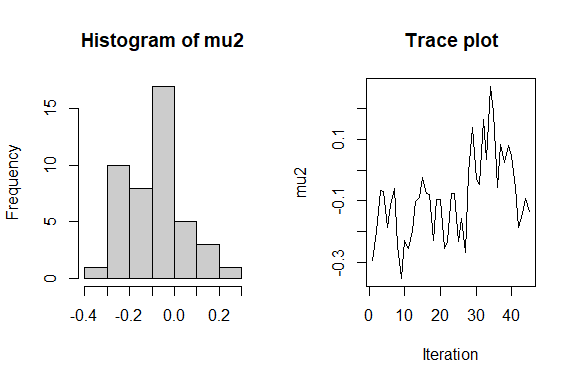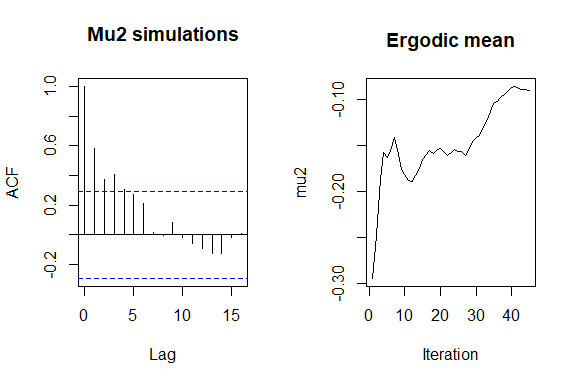# PPTCirc User Guide.

This vignette will describe the use of the package PPTcirc and the mathematical theory behind the model Projected Polya Tree of Nieto-Barajas and Nunez-Antonio (2021). Journal of Computational and Graphical Statistics. To be published.

library(PPTcirc)

First, this package is build specially for circular data. Circular data is two-dimension directional data. Directional data arise from the observation of unit vectors in k-dimensional space and can be represented through k-1 angles. The simplest way to define a circular distribution on $$\mathbb{S}^2$$ is to radially project probability distributions originally defined in $$\mathbb{R}^2$$. The model projected Polya tree of Nieto-Barajas and Nunez-Antonio (2021) is based on a projected Polya tree centered on a projected Normal model.

## Projected Polya tree

Polya trees are a non-parametric distribution and can be described as random histograms, with a fixed number of classes or partitions and with a random probability mass associated with each one.

Their advantage lies in the fact that they can be constructed in such a way as to give probability one to the set of continuous or absolutely continuous probability measures. For the mathematical definition see: Nieto-Barajas and Nunez-Antonio (2021) https://arxiv.org/abs/1902.06020. Polya trees can be centered around a parametric probability measure $$F_0$$. In this model, we will match the partition with the dyadic quantiles.

For the case of a bivariate Polya tree, we define the partition as the cross product of univariate partitions. In the model, the bivariate Polya tree is centered around a parametric probability measure $$F_0$$. For simplicity, let us assume that $$F_0(x_1; x_2) = F_{1_0}(x_1)F_{2_0}(x_2)$$ defines a bivariate distribution on $$\mathbb{R}^2$$. Therefore, we proceed as in the univariate case by matching the partition with the dyadic quantiles of the marginals $$F_{1_0}$$ and $$F_{2_0}$$.

As we explained, we can project a bivariate distribution on $$\mathbb{R}^2$$ into a circular distirbution. We construct the projected Polya tree. Let us assume a bivariate random vector $$X_0 = (X_1,X_2)$$ such that $$X \sim f$$. We project the random vector $$X$$ to the unit circle by defining $$U = X/||X||$$, or by using polar coordinates. In this model all the prior projected Polya trees will be centered on the projected Normal distribution .

### Prior projected Polya Tree in the package

The function dsimpriorpppt() simulates paths of prior projected Polya tree distributions centered around a projected Normal distribution.

The main parameters are:

• nsim: integer indicating the number of simulations.
• mm: integer indicating the number of finite levels of the Polya tree.
• mu: mean vector of the projected bivariate normal distribution.
• units: units of the support: “radians”, “degrees” or “hours”.

There are other functions that allows us to visualize and summarize the Polya tree distribution:

1. priorppt.plot: plots a linear or circular of the projected Polya paths, or a boxplot of the circular mean and concentration of the simulated paths, depending of the value of the parameter plot.type.
2. priorppt.summary: returns a table with the mean, quantiles 2.5% and 97.5% of the mean direction and concentration.
z1 <- dsimpriorppt(nsim = 10, mm = 4,  mu = c(0,0), sig = 1, ll = 100,
aa = 1, delta = 1.1, units = "radians")
class(z1)
#>  "priorppt.circ"
priorppt.plot(z1, plot.type = "line")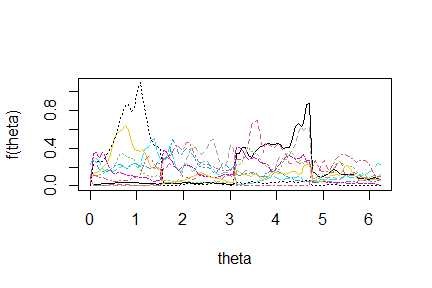priorppt.plot(z1, plot.type = "circle", tol = 8, shrink = .13)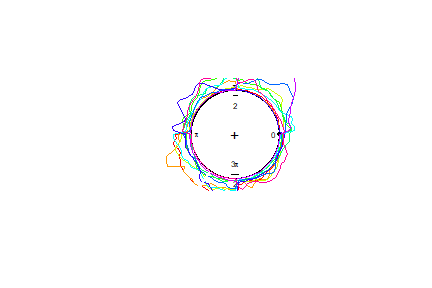priorppt.summary(z1)
#>              mean.direction concentration
#> mean              2.3388108     0.4111898
#> quantile2.5       0.6942642     0.1909665
#> quantile97.5      4.6798731     0.7481354

Depending of the value of the mean vector of the centered projected bivariate normal distribution, the paths will change, the location parameter of the centering measure not only will control the shape of the densities but also the variability of the paths. The larger the magnitude of mu, the greater the concentration:

mat_mu <- matrix(c(0,0,-1,0,0,1,2,2), nrow = 4, ncol = 2, byrow = TRUE)
for(i in 1:4){
z <- dsimpriorppt(nsim = 10, mm = 4,  mu = mat_mu[i,], sig = 1, ll = 100,
aa = 1, delta = 1.1, units = "radians")
priorppt.plot(z, plot.type = "line")
}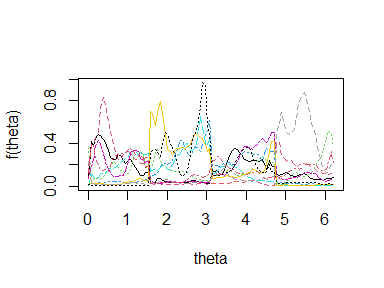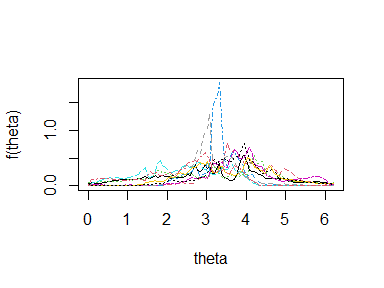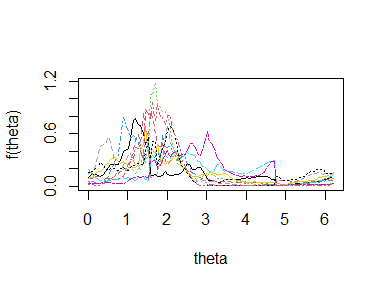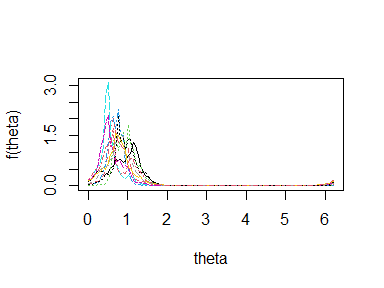## Bayesian Inference with posterior Projected Polya Tree

Given a sample of circular data $$\theta_1, \theta_2, ..., \theta_n$$ of size $$n$$, the model of Nieto-Barajas and Nunez-Antonio (2021) obtains the predictive posterior distribution via Markov Chain Monte Carlo methods: Gibbs Sampler and Metropolis Hastings. The implementation of the model is done with the function dsimpostppt and returns an object with class postppt.circ whose underlying structure is a list with main components:

• predictive: predictive density estimated with the projected Polya tree.
• quantile2.5 & quantile97.5: lower and upper 95% credible interval limits.
• stats: descriptive statistics: mean direction and concentration of each MCMC density.
• LMPL: logarithm of the pseudo marginal likelihood statistic to compare models.

The function dsimpostppt is able to make the posterior inference for your specific data file varying the number of finite levels of the Polya tree or the mean vector of the projected bivariate normal centering distribution, with the respective parameters mm and mu. For more detail of the parameters see the documentation of the function dsimpostppt.

### Posterior Projected Polya Tree Inference in the package

Tha package PPTcirc has already three circular datasets that reports temporal activity information (time of the day in radians) when a camera detected the appearance three different animals (deer, peccary and tapir) at El Triunfo biosphere in Mexico in 2015. For this example we will work with the peccary data.

peccary <- data("peccary")

The function is flexible to not only choose different values for $$\alpha$$ (alpha), but to place a hyper-prior distribution, $$\alpha \sim Ga(c_\alpha, d_\alpha)$$, and update it with its corresponding conditional posterior distribution, obtaining draws with a MH step.

Additionally, further smoothing can be achieved if we also assign a hyper-prior distribution to the location parameter $$\mu$$ (mu) of the projected normal centering measure $$f_0$$, inducing a mixture in the nested partitions.

For the first procedure we need to set ha=TRUE and for the second, hm=TRUE. Note we can do both at the same time.

We will create two models and compare them. For this example we will use only 100 iterations, 10 burn-out and the parameter for thinning as 2. We strongly recommend to use around 10,000 iterations but only for this illustration example we will use 100 iterations.

The first model will be run as default and the second model will assign prior distributions to $$\alpha$$ alpha and $$\mu$$ mu.

set.seed(2021)
rm(list=ls())
model_1 <- dsimpostppt(peccary, units = "radians", mu = c(0,0),
it = 100, ti=2, bi=10)
model_2 <- dsimpostppt(peccary, units = "radians", mu = c(0,0),
it = 100, ti=2, bi=10, ha = 1, hm = 1)
postppt.plot(model_1, plot.type= "line" , ylim = c(0,0.8))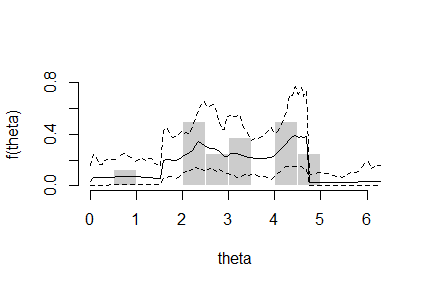postppt.plot(model_2, plot.type= "line" , ylim = c(0,0.8))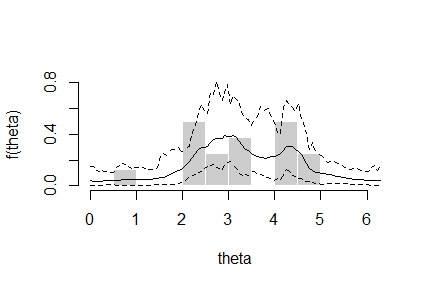To compare models we will use the LMPL statistic. The biggest value will have a better model fit. In this case, the first model had a better fit, but the second model is smoother.

model_1$LMPL #>  -23.19224 model_2$LMPL
#>  -25.98433

The package allow also to describe summary statistics for the posterior distribution. The $$95\%$$ credible interval of the mean direction and concentration are from 3.22 to 3.8 and 0.46 to 0.63, respectively. Anoter way to show this is with the function postppt.plot with the parameter


postppt.summary(model_1)
#>              mean.direction concentration
#> mean               3.191442     0.4644355
#> quantile2.5        2.488255     0.2523215
#> quantile97.5       3.751529     0.6312218
postppt.plot(model_1, plot.type = "summary")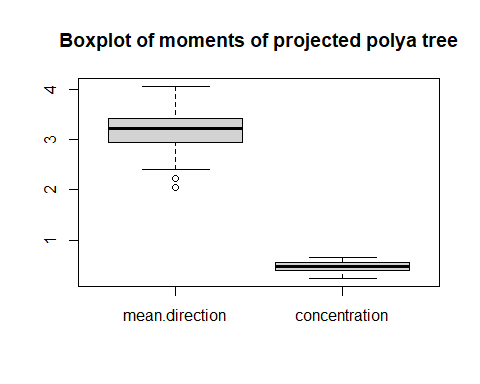Finally, the functions of the package allow to make an assessing Convergence of the Markov Chain Monte Carlo of alpha and mu in order to determine if the chain has converged or not. The function postppt.plot with the parameter plot.type set as a.sim or mu.sim display four graphs for the simulated values of alpha and mu, respectively. These graphs are:

1. Histogram of simulated values
2. Trace plot
3. Autocorrelated Function
4. Ergodic mean
postppt.plot(model_2, plot.type = "a.sim" )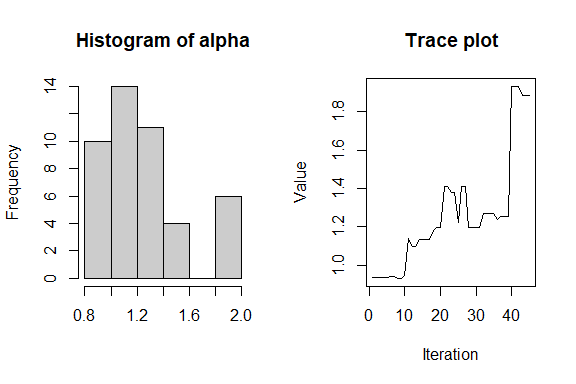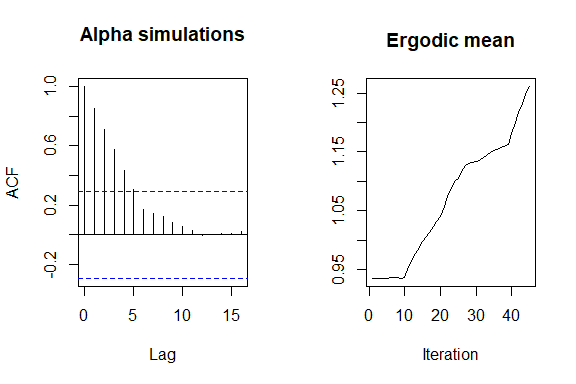postppt.plot(model_2, plot.type = "mu.sim")